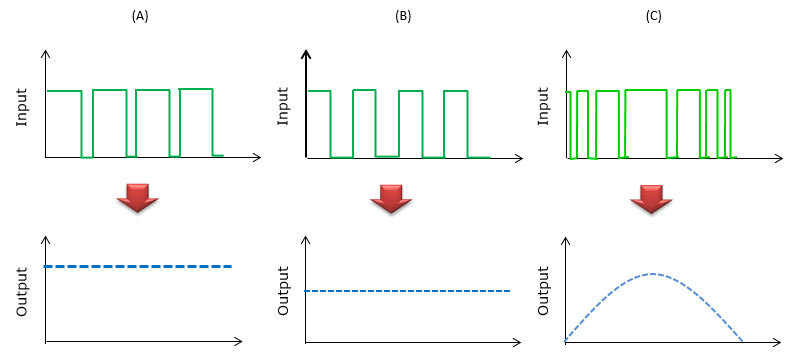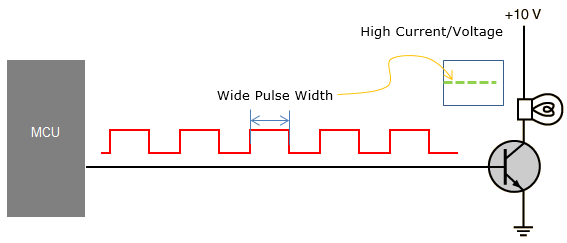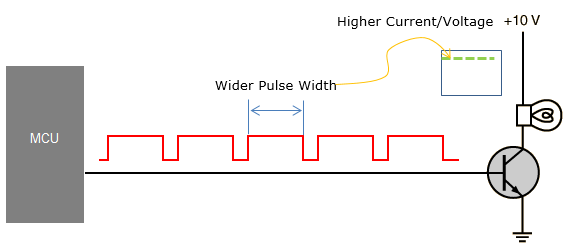EmbeddedSystem - Microcontroller - PWM                             Home : www.sharetechnote.com PWM stands for Pulse Width Modulation. 'Modulation' means 'Converting one thing to another'. So PWM would mean 'Converting Pulse Width to another thing'. What is the 'another thing' here ? In PWM case, the 'another thing' means 'Amplitude'. Therefore, PWM mean 'Converting the pulse width to the amplitude'.   Take a look at some of the examples as illustrated below. In (A), you see a sequence of 'ON'/'OFF' repetition but ON Width is wider than OFF width. However, every ON has the same width and every OFF has the same width. If this is converted to amplitude by PWM, you would get the relatively high amplitude as shown in the dotted line at the bottom. In (B), you see a sequence of 'ON'/'OFF' repetition but ON Width is almost the same as OFF width. And, every ON has the same width and every OFF has the same width. If this is converted to amplitude by PWM, you would get the relatively low amplitude comparing to (A) as shown in the dotted line at the bottom. In (C), you see a sequence of 'ON'/'OFF' repetition but ON Width and OFF width varies at every pulse.  If this is converted to amplitude by PWM, you would get gradually changing amplitude as shown at the bottom plot.I hope you got the big picture of PWM. Then you may ask "Why we use this technique ?" or "When do we use this kind of technique ?".   In short, the answer is "Using PWM, you can convert a digital pulse with has only two states (ON or OFF) into any arbitrary level of amplitude'. So, in real application, the input is almost always digital pulse (DC voltage or DC current) and output becomes various other forms depending on the application.   Following is one of the simplest example of using PWM and showing the concept of PWM. Using the train of digital pulse with various different pulse width and produce the effect of different brightness of a light (analog concept).As other examples, if you use PWM for DC motor control, the 'input' might be digital signal pulse and the output becomes the speed of the motor spin (Refer to  "How to Change the speed of the motor spin ?") As another example, you can implement DC-DC converter to use PWM.